# Set Relations

A relation (“ is Religion of”) between SET A to SET B is a sub set of A×B
Where ‘R ≤ A×B
If SET A & SET B is not Vol D SET & If (a,b)R read as a is related to b by relation R’ if (a, b) R then a ×b (a is not related to b & y any relation R
Total Number Of Relation: If SET A and SET B not empty finite sets consisting of m and n elements then A×B consists of mn ordered pairs and total no. of sub sets of A×B is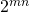Among theserelations the void relation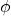& the universal relation A×B are trivial relations from A to B.

Domain And Range Of A Relation– relation to SET A to SET B all first components or co ordinates of the ordered pairs belonging to R is called the domain while the set of all second components or co-ordinates of the ordered pair in the R is called the range of R.

Relation On A Set– A non raid SET- A to relation is set (SET A) = A×A is called a relation on SET A
Inverse relation:- If SET A and SET B two sets and R is relation between them then inverse of R denoted by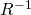, is a relation from B to A= {(b,a): (a,b)R}

Functions: a relation F from SET A to SET B i.e a subset of A×B is called function L or a mapping (or a map) from A to B if

(i) For each aA there exists bε B such that (a,b) f
(ii) (a,b)f and (a,c)f b=c

A non void set f of A×b is a function from A to B if each elements of A appears in some ordered pairs in f and no two ordered pairs in f have the first element.

If (a,b)f then b is called the image of a under f.
e.g. set A= (2,3,4)
set B = (3,4,5)
f1, f2, f3 is three sub set of A×B as below.
f= {(1,2,3), (3,4), (4,5)}
f2 = {(2,3), (2,4) (3,4) (4,5)}
f3= {(2,4), (3,4)}
here f1 is a function from A to B
f2 is not a function from A to B because 2A has two images 3 and 4 in B.
f3 is not a function A to B because 4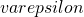A has no image in B.
if a function F is expressed as the set of ordered pair, the domain of f is the set of all first component(elements ) of member s of f & the range of f is the set of second components of member of f.

Function As A Correspondence
If A and B is non empty sets, then a function f from set A to set B is a rule or method or correspondence. Which associate elements of set A to set B such that
(1) All elements of Set A are associated to elements in set B.
(2) An element of set A is associated to a unique element in set B.
If ‘ f ’is a function from a set A to set B then we write f:AB or A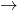B and read as “f is a function from A to B”.
If an elements aA is associated to an elements bε B then B is called the f image of a’ or image of a under f’ or the value of the function f at a’

A is called the pre image of B under function f & write b = f(a)

Description of a function:- if f:AB be a function such that the set A consists of a finite number of elements than f(x) described by listing the values it attains at different paints of its domain

Domain Co Domain And Range Of A Function:
IF f :AB , then the set A is known as the domain of f and the set B is known as the co domain of f. The set of all of image of elements of A is known as the range of f or image set of A under f and is denoted by f(A)

F(A)= {f(x): xA} = range of f or f(A) ≤ B
e.g. Set A= {-2,-1,0,1,2,} and B= {0,1,2,3,4,5,6}
Consider a rate f(x) =then f(-2) =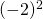= 4
f(-1) =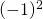= 1
f(0) =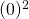= 0
f(1) =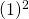= 1
f(2) =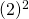= 4

as above each elements of A is associated to a unique elements of B so,is given by f(x) =is a function

Here domain (f) = A {-2,-1,0,1,2}
Range (f) = {0,1,4}

EQUAL FUNCTION
Two functions f & g are said to be equal, if
(1) Domain of f = domain of g.
(2) Codomain of f = co-domain of g
(3) F(x) = g(x) for every x beginning to their common domain then function f = function g

Real Function : if domain and co domain are sub set of :
(A) (B)
The set R of all real number , function are called real function.
Domain of real function:- real functions are described. By providing the general for mald for finding the image of elements in its domain.

Range Of Real Function:- the range of a real function of a real variable. Is the set of all real value fallen by f(x) at paints in its domain.
Constant Function:- if K is a fixed real number
Then f(x) = k (xR)
The graph of a constant function f(x) = K is a straight line. Parallel of x – axis above or below of x axis according positive or negative value of K, if k = 0 the straight line is coincident of x axis

Set identity functions